# Troops

The route is long 147 km and the first-day first regiment went at an average speed of 12 km/h and journey back 21 km/h. The second day went second regiment the same route at an average speed of 22 km/h there and back.

Which regiment will take route longer?

Result

#### Solution: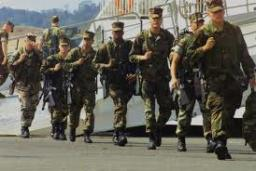Our examples were largely sent or created by pupils and students themselves. Therefore, we would be pleased if you could send us any errors you found, spelling mistakes, or rephasing the example. Thank you!

Please write to us with your comment on the math problem or ask something. Thank you for helping each other - students, teachers, parents, and problem authors.

Showing 1 comment:Math student
3qne=b=3wn8e b=Tips to related online calculators
Need help calculate sum, simplify or multiply fractions? Try our fraction calculator.
Check out our ratio calculator.
Do you have a linear equation or system of equations and looking for its solution? Or do you have quadratic equation?
Do you want to convert length units?
Do you want to convert velocity (speed) units?
Do you want to convert time units like minutes to seconds?

## Next similar math problems:

• Divide in ratioLine segment AB 12 cm long divide in a ratio of 5: 3. How long are the individual parts?
• Fountain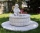The stone fountain, which has the shape of a cylinder with a diameter of 3 m, is 70 cm deep. How many m2 of stone is wetted with water?
• Shoemaker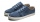Both the shoemaker and his apprentice repaired his shoes. The apprentice worked for 6 days and repaired 10 pairs of shoes every day. The shoemaker did the same job in 4 days. How many pairs of shoes did the shoemaker repair per day?
• Cylinder containerThe cylindrical container with a diameter of 1.8 m contains 2,000 liters of water. How high does the water reach?Calculate the volume of a quadrilateral pyramid, the base of which has the shape of a rectangle with dimensions a = 6cm, b = 4cm and height v = 11cm
• The trench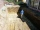Calculate how many cubic meters of soil needs to be removed from the excavation in the shape of an isosceles trapezoid, the top width is 3 meters, the lower width is 1.8 m, the depth of the excavation is 1 m, and the length is 20 m.
• Empty barrel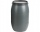In the backyard is an empty barrel with a volume of 2 hl. How many liters are missing at the edge, when 53 liters flowed into it for 1 day and 68 liters of water for 2 days?
• Largest wallFind the content of the largest wall of a prism with the base of a rectangle which has a height of 4 dm, side c = 5 cm, and side b = 6 cm.
• The plan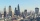The plan of the housing estate is in three scales 1: 5000,1: 10000,1: 15000. The distance between two points on a plan with a scale of 1: 10000 is 12 cm. What is this distance on the other two plans? What is this distance?Calculate the surface of a quadrilateral prism according to the input: Area of the diamond base S1 = 2.8 m2, length of the base edge a = 14 dm, height of the prism 1,500 mm.How many times does the surface of a sphere decrease if we reduce its radius twice?The sum of five consecutive odd numbers is 75. Find out the sum of the second and fourth of them.25 tons of old paper will save 1,600 trees. How many tons of paper is needed to save the 32 trees in the park?How high does the water in the aquarium reach, if there are 36 liters of water in it? The length of the aquarium is 60 cm and the width is 4 dm.Calculate the volume of the cube if its surface is 150 cm2.Josh is at Enzo's pizza palace. He can sit at a table with 5 of his friends or at a different table with seven of his friends. The same size pizza is shared equally among the people at each table. At which table should Josh sit to get more pizza? (write aThe concrete hatch for a round well has a diameter of 1300 mm and a thickness of 80 mm. Determine its weight in kg if the density of the concrete is 2545 kg/m3